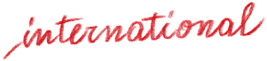# Happy Mathematics – Year Four

Worksheets, games and activities for primary school

Antonella Fedele, Antonio Saltarelli

Product: Book

Trim size in cm: 21x29,7cm

Pages: 296

ISBN: 978-88-590-1879-7

Publication date: 01/05/2019

Suitable for: Primary 2nd level (ages 8-10)

Like in previous volumes in the series, Happy Mathematics- Fourth Grade teaches mathematics in a fun and narrative way, which helps children approach the topic with calmness and curiosity.

Along with Cloud, Mistral, Mitzy, Mizar and Balù, the students participate in activities which require them to classify, compose and decompose numbers, compare them, order them, use different calculation strategies, recognize the properties of operations, solve riddles and word problems in everyday contexts, work with fractions, decimal numbers, units of measurement, and geometric shapes.  Each situation presented favours the acquisition of the skills necessary to analyze, understand and make the correct choices, as well as the translation of skills into mathematical skills.

Aimed at primary school teachers, this volume can also be used at home to practice
• Sets, subsets and natural numbers
• The decimal and positional numbering system
• The four operations and their properties
• Expressions
• Fractions
• Word problems
• Measurements of length, weight, capacity, time
• Elements of statistics, Cartesian diagrams
• Flat figures, isometries.

Introduction
Sets, subsets, natural numbers
The decimal and positional numbering system
Addition and subtraction with natural numbers, their properties, word problems
Multiplication and division with natural numbers, their properties, word problems
Expressions
Fractions
Decimal fractions (with denominators equal to a multiple of 10), decimal numbers
Decimal numbers, operations, word problems
Measurements of length, weight, and capacity
Measurements of time
Elements of statistics, Cartesian diagrams
Isometric movements
Geometric figures, 2D shapes

Thematic Areas